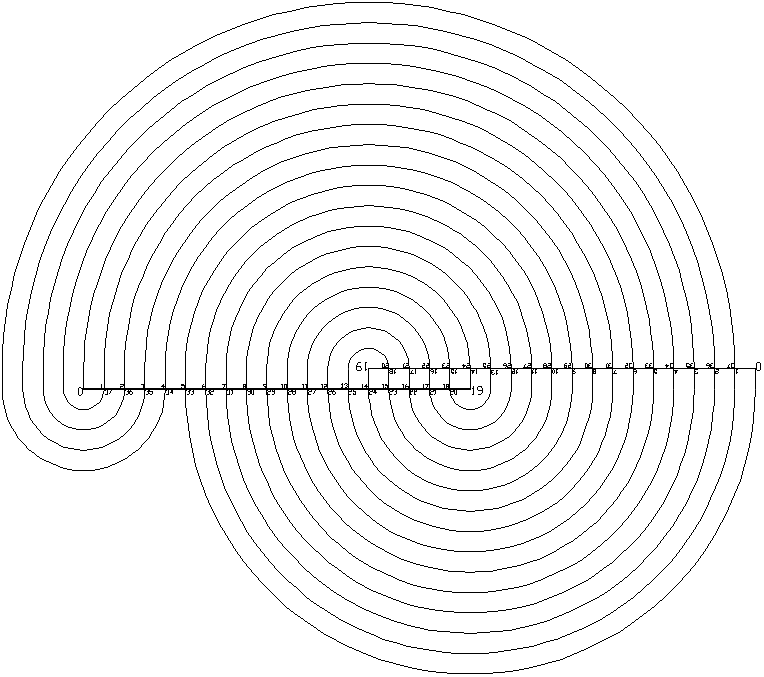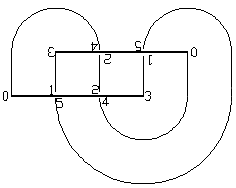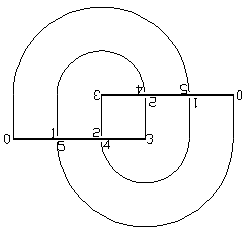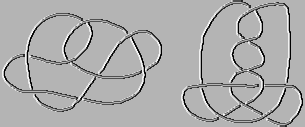This header plots the critical line of the Riemann Zeta Function.  A complete understanding wins a \$1,000,000 prize.
 . . .Main Links Orders Post Next Page Next + 10

KNOTSI borrowed A Survey of Knot Theory (by Akio Kawauchi) and Knot Theory & its Applications (by Kunio Murasugi) from the local college library this weekend, and learned a lot.  I had hoped to present a simple explanation of various knot polynomials, but I'm not sure that's possible.  Bridge representations are fairly easy to explain, though.  Each knot or link has a minimal bridge number.  A bridge is a section that goes over other sections.  The above representation of the Borromean Rings has three bridges.  Many knots require only two bridges, though.  In 1949, mathematician H. Schubert presented what is now known as Schubert's normal form.  The following knot is S(19,5).Again, the above knot is S(19,5).  The 19 comes from the length of each bridge.  I started with a line, then divided it so that I could number the ends with 0 and 19.  I also numbered the undercrossings, first 1-18 and then 20-37.  Then I copied this bridge elsewhere, upside down.  That explains the 19 in S(19, 5).  What about the 5?  Well, start with 0, and start travelling.  Note the first number you meet after passing under a bridge.  You'll find the sequence of numbers is 0, 5, 10, 15, 20, 25, 30, 35, 2, 7, 12, 17, 22, 27, 32, 37, 4, 9, 14, 19.  At every bridge crossing, your number will increase by 5, modulo 38 (A clock face can be considered modulo 12.  5:00 + 13 hours = 6:00).  The bridges always alternate.  Drawing S(89,34) would need two bridges of length 89, and a winding path that aimed at 0, 34, 68, 102, 136, 170, 26, 60, etc.  Here is what S(19,5) looks like with a minimal crossings diagram:A fantastic diagram of many simple knots is available here from the excellent KnotPlot site.  The following is my picture of Knot 85 (this is the catalog number).  This knot requires three bridges.  Is there a standardized way to represent 3-bridge knots?  This is an unsolved problem in mathematics.The Schubert normal form for 2-bridge knots by their Catalog Number

 41 = S(5,2) 52 = S(7,3) 61 = S(9,4) 62 = S(11,4) 63 = S(13,5) 72 = S(11,5) 73 = S(13,4) 74 = S(15,4) 75 = S(17,7) 76 = S(19,7) 77 = S(21,8) 81 = S(13,6) 82 = S(17,6) 83 = S(17,4) 84 = S(19,5) 86 = S(23,10) 87 = S(23,9) 88 = S(25,9) 89 = S(25,7) 811 = S(27,10) 812 = S(29,12) 813 = S(29,11) 814 = S(31,12) 92 = S(15,7) 93 = S(19,6) 94 = S(21,5) 95 = S(23,6) 96 = S(27,5) 97 = S(29,13) 98 = S(31,11) 99 = S(31,9) 910 = S(33,10) 911 = S(33,14) 912 = S(35,13) 913 = S(37,10) 914 = S(37,14) 915 = S(39,16) 917 = S(39,14) 918 = S(41,17) 919 = S(41,16) 920 = S(41,15) 921 = S(43,18) 923 = S(45,19) 926 = S(47,18) 927 = S(49,19) 931 = S(55,21)

Pictures of all of these knots can be seen in the CRC Encyclopedia of Mathematics under the knot listing.  Two knots not listed above are S(3,2) and S(3,1).  I show pictures of them below.  Can you figure out what these knots are?  Send answer.  From these, you can see that S(a,b) = S(c,d) is possible.  What are the rules for (a,b,c,d)?  What is the minimal crossing number for S(2003, 541)?  Is a smaller bridge possible for S(2003, 541)?  It would be interesting for a computer to crank through all 2-bridge knots with bridges of length 1000 or less, calculate the Alexander, Jones, and HOMFLY polynomials for each, and sort through them for equivalencies.Why so many polynomials?  The following two knots have identical Jones polynomials (-t-3 + 2t-2 - 3t-1 + 5 - 4t + 4t2 - 3t3 + 2t4 - t5) and identical Alexander polynomials (2t-2 - 6t-1 + 9 - 6t + 2t2).  They are different knots, though.For more about knots, try searching on 'Alexander Polynomial'.  Or visit any of the links I've mentioned.